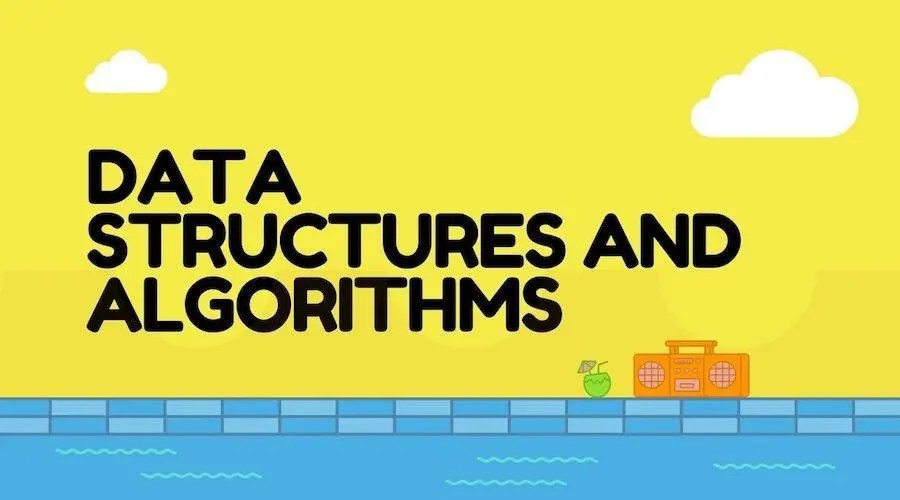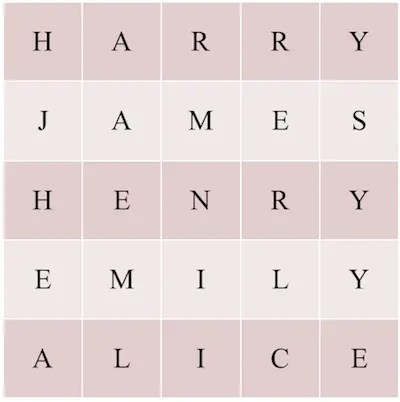# Data Structure and Algorithms | Part One Lesson 4: Algorithm Complexity (Part 2)

Posted May 27, 20207 min readAuthor Xie Enming, the public number " Programmer Union ".
Original: https://www.jianshu.com/p/3e5...

"Data Structure and Algorithm" full series

## brief introduction

1. Big O symbol
2. Time complexity and space complexity
3. The worst case complexity
4. The first part of the fifth lesson

## 1 . Big O symbol

The last lesson Data Structure and Algorithms | The first part of the third lesson:Algorithm Complexity(Part 1) We started the learning of algorithm complexity Continue to study the second half.

We have seen that complexity only considers an order of magnitude of the number of operations(ignoring other components), which is an approximation.

To express this approximation, we use a specific symbol, the famous big O symbol.

Big O notation, also known as asymptotic notation, is a mathematical symbol used to describe the asymptotic behavior of functions. More precisely, it uses another(usually simpler) function to describe the asymptotic upper bound of the order of a function.
In mathematics, it is generally used to describe the remaining terms of truncated infinite series, especially asymptotic series.
In computer science, it is very useful in analyzing the complexity of algorithms.
The big O symbol was first introduced by the German number theorist Paul Bachmann in his 1892 book Analytische Zahlentheorie. This notation was promoted in the work of another German number theorist Edmund Landau(Edmund Landau), so it is sometimes called Landau Notation.
The big O for "order of ..."(...) was originally a capital Greek letter " "(Omicron), and now it uses the capital Latin letter "O".
-Excerpt from Baidu Encyclopedia

For example, in the story of the ducklings going on vacation, the first algorithm of farmer Oscar has N2 operations. We say that the complexity of this algorithm is O(N2). Similarly, the complexity of the second faster algorithm is O(N).

The big O symbol is a bit like a big round bag, which can integrate different numbers of operations to make it have the same order of magnitude.

For example, if there are three algorithms whose number of operations are N, 5N + 7 and N/4, we all use O(N)(pronounced "N's big O". Of course, the reading is not so fixed) To represent the complexity of these three algorithms.

Similarly, if the operand of an algorithm is(2 N2 + 5 N + 7), then its complexity is O(N2):we ignore 5 \ * N and 7 because they are compared with 2N2 The order of magnitude is small. As N increases, the growth rate of these two items is slower than 2N2, so we only need to keep 2N2, and because the constant multiplication factor(here 2) is not considered, it is denoted as O(N2).

We say that f(N) means "a function of N"(for example, f(N) = 2 N2 + 5 N + 7)), then O(f(N)) means "There are about f(N) operations "The complexity of the algorithm", "approximately" here is very critical.

## 2 . Time complexity and space complexity

Let's learn about the "time complexity" and "space complexity" that are often heard in algorithms.

Why did I think of the infinite gloves of the villain in Marvel? There are two infinite gems, time gem and space gem.
It must be because I watched the relationship between "Avengers 3:Infinite Warfare" and "Avengers 4:Endgame" ...

![Six Infinite Gems on Infinite Gloves]( https://i0.wp.com/segmentfault.com/img/remote/1460000022760196 "Six Infinite Gems in Infinite Gloves"

So what do you mean by "time complexity" and "space complexity"? And listen to me slowly.

"A long time ago, there were 6 infinite gems in the universe, namely time gem and space gem ..."

Readers:"Xiaobian, you wake up and talk seriously!"
Me:"Okay, okay, serious, serious ~"

In order to express the complexity of the algorithm as accurately as possible, we can make many choices.

First, we choose the quantification of the input conditions, for example by the variable N(N rows and N columns of ducklings, N students, N planes, etc.). Of course, it is not necessary to use the variable name N, we can choose other variable names(such as M, Z, X, Y, etc.), but more importantly, we can also use more than one variable.

For example, if our problem is to draw on a piece of paper, then we may express the complexity of the algorithm as a function of the length L and width W of the drawing paper. Similarly, if the farmer Oscar has more rows of ducklings than the number of available ponds, he can express the complexity of the algorithm as a function of the number N of ducklings and the number P of ponds.

Another important choice is the type of operation to be measured. So far, we have only talked about the efficiency or performance of the algorithm(that is, whether the algorithm is fast or not). However, programmers are not only interested in the execution time of algorithms, they may also measure many other features, the most common of which is memory consumption.

Algorithm memory consumption is also a measure of algorithm complexity. For example, if you need to allocate N kilobytes(KiloByte, kilobytes, KB for short) of an algorithm with an input size of N, the memory complexity of this algorithm can be expressed as O(N).

Memory complexity is the complexity related to the memory consumption of the algorithm. It does not measure the efficiency of the algorithm, but the amount of memory space consumed/occupied. Therefore, we call it the space complexity of the algorithm(Space Complexity). In contrast, the measure of the execution speed(fast or not) of the algorithm we discussed earlier is the time complexity(Time Complexity).

Space complexity is a measure of the amount of storage space temporarily occupied by an algorithm during operation, and is recorded as S(N) = O(f(N)).
Relatively, the time complexity of the algorithm is recorded as T(N) = O(f(N)).
Because S is the first letter of Space and T is the first letter of Time.

When calculating the space complexity of an algorithm, we actually do not know the specific memory size consumed/occupied by the algorithm(memory is in bytes). We calculate the(data) structure used by the algorithm Of the order of magnitude.

For example, if you use N arrays of size N, the space complexity is O(N2).

For example, for the story of the ducklings going on vacation, maybe the farmer Oscar gave each of his ducklings an English name. He carried a form of duckling's name with him to avoid forgetting.The above table is an intuitive representation of the form used by farmer Oscar to record the names of ducklings:there are 5 names(HARRY, JAMES, HENRY, EMILY, ALICE), corresponding to 5 ducklings. Each row in the table stores a name, each row has 5 grids(similar to the 5 elements of the array), 5 x 5 = 25 grids, and one grid is an English character.

If the memory level of the computer is connected, N ducklings need N arrays to store their names. Each array contains the name of a duckling(all English characters), and the size of the array(here is the number of characters) All are unified as N. So the space complexity here is O(N2).

Sometimes, we need to consider both the time complexity(execution speed) and space complexity(the amount of memory space occupied during execution) of the algorithm.

Generally speaking, in relatively simple cases, we do not pay much attention to the space complexity of the algorithm. But for more complex problems, the space complexity of the algorithm may attract more attention:for example, we may use less memory by sacrificing a little execution speed; or even increase the execution speed by increasing the space complexity of the algorithm , For example, by storing already calculated results in a table(the principle of cache).

The more constraints on the program, the more precise the required information. In some areas of computer science, we will also be interested in other features of algorithms. And some of these features can also be measured with a certain complexity of the algorithm. For example, programmers of mainframe computers or embedded systems may consider the power consumption of algorithms to save power.

However, in general, we only focus on the time complexity and space complexity of the algorithm, and even focus mainly on time complexity.

## 3 . Worst-case complexity

As we said before, the number of operations performed by the algorithm obviously depends on the starting conditions.

For example, the following is a very simple algorithm for knowing whether a given value is in a list of values (for example, "Did I add an egg to my shopping list?"):

``````To know whether a given value is in the list of values, we can do this:
Traverse the entire list and stop when it finds a given value, indicating that the value is in the list;
If we have traversed the entire list and still do not find the given value, then the given value is not in the list of values.``````

Imagine if the value we are looking for is not in the list and there are L elements in the list. Then to determine whether this value exists, the algorithm must traverse the entire list and compare each value with the value to be searched, which will require L comparisons. Therefore, we can say that the algorithm has O(L) complexity(obviously, time complexity is considered here). We can also say that the time complexity of this algorithm is linear(if we double the size of the input list, then this algorithm will take twice as much time).

But what if the value you are looking for is at the very beginning of the list?

For example, if "egg" is the first element in our shopping list, it will be noticed immediately, and we will stop traversing after only one operation. In other cases, even if the list contains 3000 elements, our search may stop after 4 to 5 operations.

This is where the concept of "worst case" comes into play:when calculating the complexity of an algorithm, it can be assumed that a given input is in the "worst case" for our algorithm. We will calculate the number of operations in the input case that requires the most operations(not just one or two), for example, if the given value is not in the list.

From the programmer's point of view, this is a kind of security:the calculated complexity is at the "worst case", so he knows that the algorithm will only perform better.

Just as programmers in the field of cybersecurity will ask themselves "what text might the most malicious user enter to invade my website?" Questions such as urging themselves to improve the security of the application, people who focus on algorithm research I also want to know "Which element in the algorithm took most of my algorithm?"

This method can measure the so-called "worst case complexity".

In this tutorial, unless explicitly stated, we only consider the complexity of the algorithm in the worst case.

## 4 . The first part of the fifth lecture

Finally, the complexity of the algorithm is almost explained, it is really not easy. Everyone has worked hard.

Today's class is here, come on!

My name is , the public number "[Programmer League]( https://github.com/frogoscar/ProgrammerLeague/blob/master/qrcode . "jpg)" operator, elite lecturer Ms. Oscar , a lifelong learner.
Love life, like swimming and have a little understanding of cooking.
Life motto:"Go straight to the benchmark"